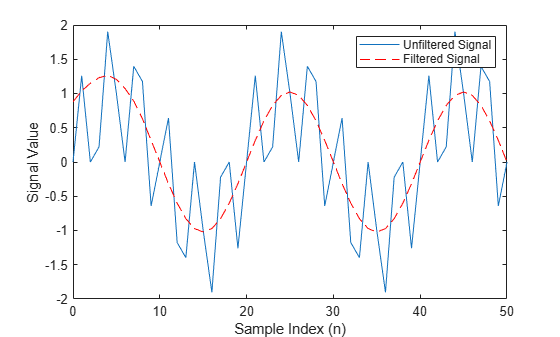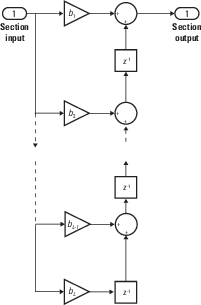# filter

One-dimensional digital filter of `fi` objects

## Syntax

```y = filter(b,1,x) [y,zf] = filter(b,1,x,zi) y = filter(b,1,x,zi,dim) ```

## Description

`y = filter(b,1,x)` filters the data in the fixed-point vector `x` using the filter described by the fixed-point vector b. The function returns the filtered data in the output `fi` object `y`. Inputs `b` and `x` must be `fi` objects. `filter` always operates along the first non-singleton dimension. Thus, the filter operates along the first dimension for column vectors and nontrivial matrices, and along the second dimension for row vectors.

```[y,zf] = filter(b,1,x,zi) ``` gives access to initial and final conditions of the delays, `zi`, and `zf`. `zi` is a vector of length `length(b)-1`, or an array with the leading dimension of size `length(b)-1` and with remaining dimensions matching those of `x`. `zi` must be a `fi` object with the same data type as `y` and `zf`. If you do not specify a value for `zi`, it defaults to a fixed-point array with a value of `0` and the appropriate `numerictype` and size.

`y = filter(b,1,x,zi,dim)` performs the filtering operation along the specified dimension. If you do not want to specify the vector of initial conditions, use `[]` for the input argument `zi`.

## Input Arguments

 `b` Fixed-point vector of the filter coefficients. `x` Fixed-point vector containing the data for the function to filter. `zi` Fixed-point vector containing the initial conditions of the delays. If the initial conditions of the delays are zero, you can specify zero, or, if you do not know the appropriate size and `numerictype` for `zi`, use `[]`. If you do not specify a value for `zi`, the parameter defaults to a fixed-point vector with a value of zero and the same `numerictype` and size as the output `zf` (default). `dim` Dimension along which to perform the filtering operation.

## Output Arguments

 `y` Output vector containing the filtered fixed-point data. `zf` Fixed-point output vector containing the final conditions of the delays.

## Examples

collapse all

The following example filters a high-frequency fixed-point sinusoid from a signal that contains both a low- and high-frequency fixed-point sinusoid.

```w1 = .1*pi; w2 = .6*pi; n = 0:999; xd = sin(w1*n) + sin(w2*n); x = sfi(xd,12); b = ufi([.1:.1:1,1-.1:-.1:.1]/4,10); gd = (length(b)-1)/2; y = filter(b,1,x); % Plot results, accommodate for group-delay of filter plot(n(1:end-gd),x(1:end-gd)) hold on plot(n(1:end-gd),y(gd+1:end),'r--') axis([0 50 -2 2]) legend('Unfiltered signal','Filtered signal') xlabel('Sample index (n)') ylabel('Signal value')```The resulting plot shows both the unfiltered and filtered signals.

collapse all

### Filter length (L)

The filter length is `length(b)`, or the number of filter coefficients specified in the fixed-point vector `b`.

### Filter order (N)

The filter order is the number of states (delays) of the filter, and is equal to `L`-`1`.

## Tips

• The `filter` function only supports FIR filters. In the general filter representation, b/a, the denominator, a, of an FIR filter is the scalar 1, which is the second input of this function.

• The `numerictype` of `b` can be different than the `numerictype` of `x`.

• If you want to specify initial conditions, but do not know what `numerictype` to use, first try filtering your data without initial conditions. You can do so by specifying `[]` for the input `zi`. After performing the filtering operation, you have the `numerictype` of `y` and `zf` (if requested). Because the `numerictype` of `zi` must match that of `y` and `zf`, you now know the `numerictype` to use for the initial conditions.

## Algorithms

collapse all

The `filter` function uses a Direct-Form Transposed FIR implementation of the following difference equation:

`$y\left(n\right)={b}_{1}*{x}_{n}+{b}_{2}*{x}_{n-1}+...+{b}_{L}*{x}_{n-N}$`

where `L` is the filter length and `N` is the filter order.

The following diagram shows the direct-form transposed FIR filter structure used by the `filter` function:### fimath Propagation Rules

The `filter` function uses the following rules regarding `fimath` behavior:

• `globalfimath` is obeyed.

• If any of the inputs has an attached `fimath`, then it is used for intermediate calculations.

• If more than one input has an attached `fimath`, then the `fimaths` must be equal.

• The output, `y`, is always associated with the default `fimath`.

• If the input vector, `zi`, has an attached `fimath`, then the output vector, `zf`, retains this `fimath`.

## Version History

Introduced in R2010a